matplotlib is the workhorse of data science visualization. The module pyplot gives us MATLAB like plots.

The most basic plot is done with the “plot”-function. It looks like this: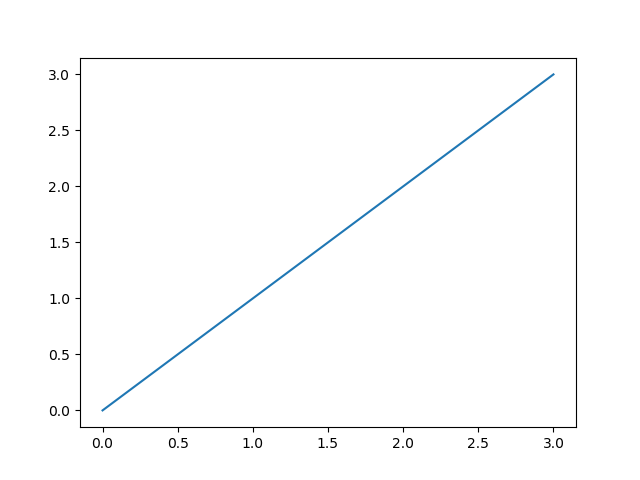```import matplotlib.pyplot as plt
plt.plot([0, 1, 2, 3], [0, 1, 2, 3])
plt.show()
```

The plot function takes an x and y array and draws a blue line through all points.

You can of course draw each point independently without a line:

`plt.plot([0, 1, 2, 3], [0, 1, 2, 3], "o")`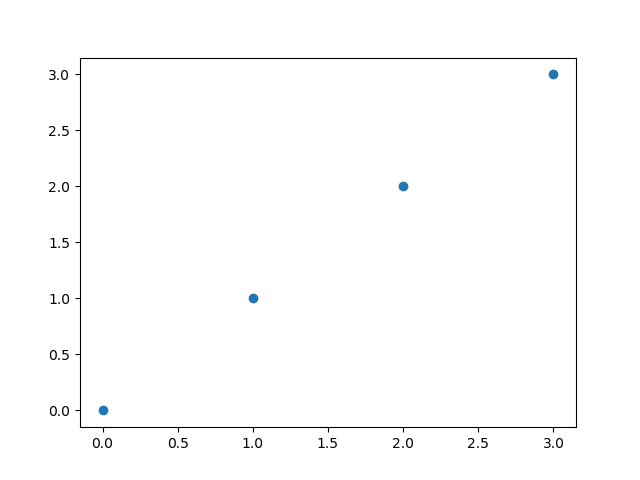or you can highlight the individual points while drawing the line

`plt.plot([0, 1, 2, 3], [0, 1, 2, 3], marker='o')`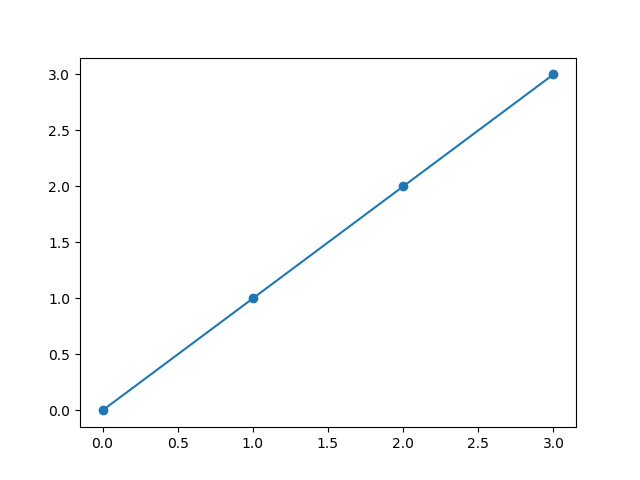You can find other markers here.

## Color of the plot

The color of the line can be changed with the color parameter. The default color of a plot is blue. If you want e.g. red as the color, you can use ‘r’.

`plt.plot([0, 1, 2, 3], [0, 1, 2, 3], color="r")`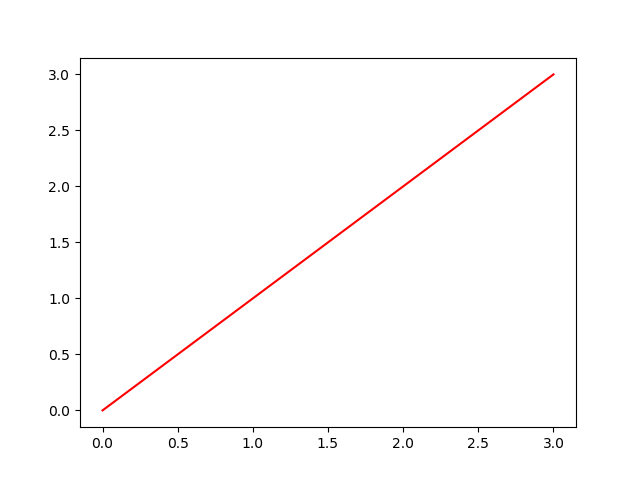The following table shows the basic colors

Color shortcut
blue b
green g
red r
cyan c
magenta m
yellow y
black k (not so obvious :-))
white w

In /matplotlib/_color_data.py you find additional colors, even colors from the XKCD color survey results

`plt.plot(x, y, color="xkcd:nasty green")`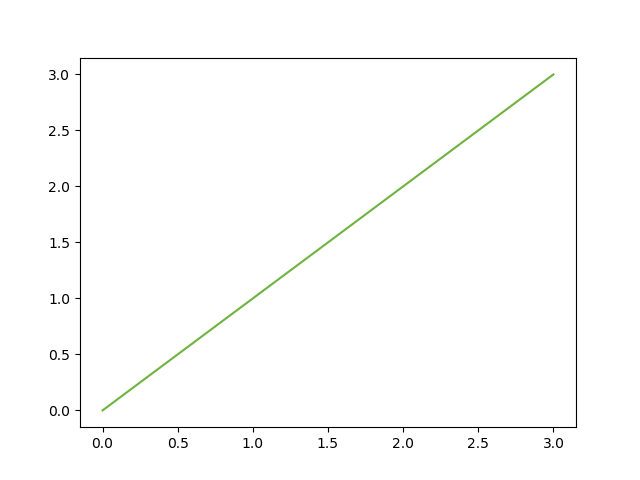## Stroke width and style

changing the width of the plotted line is done via linewidth

`plt.plot([0, 1, 2, 3], [0, 1, 2, 3], linewidth=7.0, color="xkcd:nasty green")`

##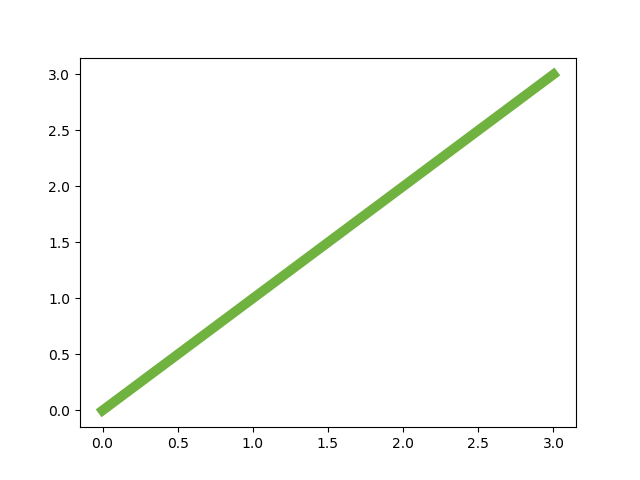and the stroke style can be altered with the linestyle parameter

`plt.plot([0, 1, 2, 3], [0, 1, 2, 3], linestyle=":", color="xkcd:nasty green", linewidth=7.0)`

##You can find other line styles here

## Axis Labels

In school I learned that all axis of a plot must have labels. So let’s add them:

```plt.ylabel('some other numbers')
plt.xlabel('some numbers')```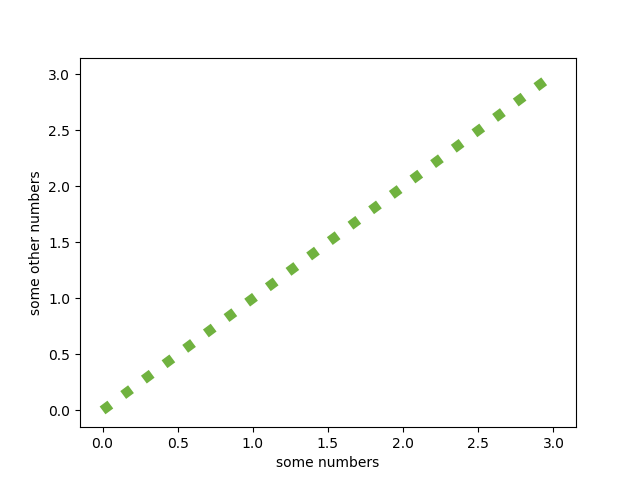## Saving the plot

If You want to save the plot as a png you can replace the show command with

`plt.savefig('scatter_01.png')`

You can find the code examples in one JuPyter notebook in my github repo

When you’ve become familiar with the basic plot function you can dive into part 2